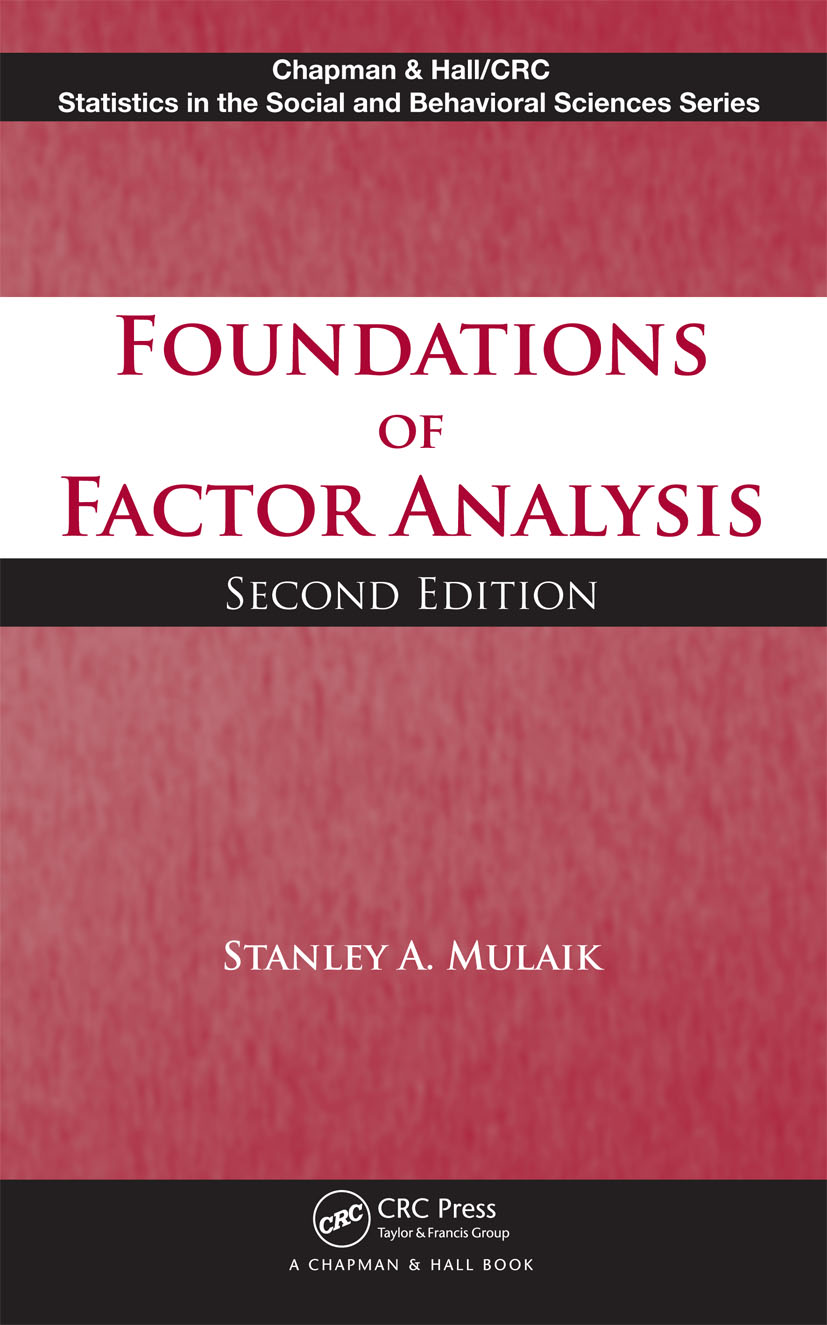Foundations of Factor Analysis

2nd Edition

Chapman and Hall/CRC

548 pages | 50 B/W Illus.

Purchasing Options:\$ = USD
Hardback: 9781420099614
pub: 2009-09-25
SAVE ~\$20.00
\$100.00
\$80.00
x
eBook (VitalSource) : 9780429132094
pub: 2009-09-25
from \$50.00

FREE Standard Shipping!

Description

Providing a practical, thorough understanding of how factor analysis works, Foundations of Factor Analysis, Second Edition discusses the assumptions underlying the equations and procedures of this method. It also explains the options in commercial computer programs for performing factor analysis and structural equation modeling. This long-awaited edition takes into account the various developments that have occurred since the publication of the original edition.

New to the Second Edition

• A new chapter on the multivariate normal distribution, its general properties, and the concept of maximum-likelihood estimation
• More complete coverage of descriptive factor analysis and doublet factor analysis
• A rewritten chapter on analytic oblique rotation that focuses on the gradient projection algorithm and its applications
• Discussions on the developments of factor score indeterminacy
• A revised chapter on confirmatory factor analysis that addresses philosophy of science issues, model specification and identification, parameter estimation, and algorithm derivation

Presenting the mathematics only as needed to understand the derivation of an equation or procedure, this textbook prepares students for later courses on structural equation modeling. It enables them to choose the proper factor analytic procedure, make modifications to the procedure, and produce new results.

Reviews

"The author does a good job in explaining basic formal and mathematical concepts. … a useful text for psychologists and scientists from other fields that deal with similar data. The book covers a wide range of topics related to exploratory factor analysis and is useful to readers interested in that topic."

Statistical Papers (2014) 55

"I must say that I am very happy that the author has taken the challenge to update and revise this precious book into the second edition. It will be an important source for decades to come. … there are good grounds for the new edition … it digs deep into the foundations of factor analysis … the topics are explained clearly, and mathematics is taught, as it is needed to understand a derivation of an equation or some procedure. … the book is worth having nearby … ."

International Statistical Review (2010), 78

Introduction

Factor Analysis and Structural Theories

Brief History of Factor Analysis as a Linear Model

Example of Factor Analysis

Mathematical Foundations for Factor Analysis

Introduction

Scalar Algebra

Vectors

Matrix Algebra

Determinants

Treatment of Variables as Vectors

Maxima and Minima of Functions

Composite Variables and Linear Transformations

Introduction

Composite Variables

Unweighted Composite Variables

Differentially Weighted Composites

Matrix Equations

Multiple and Partial Correlations

Multiple Regression and Correlation

Partial Correlations

Determinantal Formulas

Multiple Correlation in Terms of Partial Correlation

Multivariate Normal Distribution

Introduction

Univariate Normal Density Function

Multivariate Normal Distribution

Maximum-Likelihood Estimation

Fundamental Equations of Factor Analysis

Analysis of a Variable into Components

Use of Matrix Notation in Factor Analysis

Methods of Factor Extraction

Rationale for Finding Factors and Factor Loadings

Diagonal Method of Factoring

Centroid Method of Factoring

Principal-Axes Methods

Common-Factor Analysis

Preliminary Considerations

First Stages in a Factor Analysis

Fitting the Common-Factor Model to a Correlation Matrix

Other Models of Factor Analysis

Introduction

Component Analysis

Image Analysis

Canonical-Factor Analysis

Problem of Doublet Factors

Metric Invariance Properties

Image-Factor Analysis

Psychometric Inference in Factor Analysis

Factor Rotation

Introduction

Thurstone’s Concept of a Simple Structure

Oblique Graphical Rotation

Orthogonal Analytic Rotation

Introduction

Quartimax Criterion

Varimax Criterion

Transvarimax Methods

Simultaneous Orthogonal Varimax and Parsimax

Oblique Analytic Rotation

General

Oblimin Family

Harris–Kaiser Oblique Transformations

Weighted Oblique Rotation

Oblique Procrustean Transformations

Rotating Using Component Loss Functions

Conclusions

Factor Scores and Factor Indeterminacy

Introduction

Scores on Component Variables

Indeterminacy of Common-Factor Scores

Further History of Factor Indeterminacy

Other Estimators of Common Factors

Factorial Invariance

Introduction

Invariance under Selection of Variables

Invariance under Selection of Experimental Populations

Comparing Factors across Populations

Confirmatory-Factor Analysis

Introduction

Example of Confirmatory-Factor Analysis

Mathematics of Confirmatory-Factor Analysis

Designing Confirmatory Factor-Analysis Models

Some Other Applications

Conclusion

References

Subject Index

Author Index